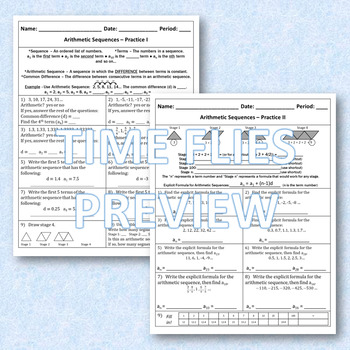DID YOU KNOW:
Seamlessly assign resources as digital activities

Learn how in 5 minutes with a tutorial resource. Try it Now

Learn More# Arithmetic Sequence Activity8th - 11th
Subjects
Standards
Resource Type
Formats Included
• PDF
Pages
21 total, 8 worksheets, 8 answer keys

### Description

This is a comprehensive look at arithmetic sequences. Each new practice page starts with a set of guided notes at the top of the page to help students understand the content. There are 4 practices, a mixed practice and a 10 question quiz. Take a look at what is contained in each piece:

•Practice I – Important terminology is introduced such as term, sequence, common difference and arithmetic sequence. The student learns how to find certain terms and how to find the common difference.

•Practice II – How to write the explicit form from various representations such as a table, word problem, diagrams and other given information and how to find a specific term.

•Practice III – More multi-reps and how to write arithmetic formulas using function notation.

•Practice IV – Writing recursive form using subscript form and function notation.

•Practice V – Mixed review of all.

•Quiz – 10 question quiz that covers all the content.

It is recommended to do each of the pages in this order. The teacher should go through the guided notes with the class and work one problem from each page to get the students started.

Related Activities:

Arithmetic Sequences Boom Cards

Geometric Sequences Lesson

Follow Me - When you follow me, you will be notified of new products which are always 50% off for 24 hours!

Total Pages
21 total, 8 worksheets, 8 answer keys
Included
Teaching Duration
2 days
Report this Resource to TpT
Reported resources will be reviewed by our team. Report this resource to let us know if this resource violates TpT’s content guidelines.

### Standards

to see state-specific standards (only available in the US).
Construct linear and exponential functions, including arithmetic and geometric sequences, given a graph, a description of a relationship, or two input-output pairs (include reading these from a table).
Write arithmetic and geometric sequences both recursively and with an explicit formula, use them to model situations, and translate between the two forms.
Determine an explicit expression, a recursive process, or steps for calculation from a context.